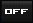View Single Post01-23-2010, 07:55 PM   #894
Initiate
Avalon Senior Member

Join Date: Aug 2009
Location: New Zealand
Posts: 391Re: Thuban Q&A: (warning longer than normal posts here)

[QUOTE=abraxasinas;227249]
Quote:
 Originally Posted by InitiateYes, NEAR 0 c* the Mathematics CAN BE refined in Metaphysical terms. The MetaMathematics of Divisibility Consider the maximum conditions of a system as being the inverse of the minimum conditions. This in a nutshell is Modular Duality as discussed in QR. Allow this LINEAR scale from minimum to maximum to RECIRCULARISE itself, so reflecting the initialising process of something NOT describable in 'measurable' terms to THEN become measurable. This is the Mathematics of 0=1=∞=Infinity as some metaphysical ultimate Identity, which 'bounds' all of the mensuration physics in principles of mathematics. The major principle here is that DIVISION DOES NOT EXIST. So one cannot divide 0 nor 1 nor ∞=Infinity. Then the Identities 0=0 and 1=1 and ∞=∞ form the SELFINTERACTION of the UNDIVIDED system. The link to DIVISION and partitioning of the UNITY in THREE (Circular closure symbolised by cipher '0' ; its unfolding symbolised by cipher '1' and its multiplication symbolised by cipher '8' as 'two circles' moebian-connected in inventing 3D from 2D and 1D and 0D*) then are the ASYMPTOTES or LIMITS of the ratios 0/0=1/1=∞/∞. {That is 3D-Volume as locus of a surface-point around a centre is contained within a 2-Sphere, whose 2D-Surface-Area is the locus of a circle as a 1-Sphere, rotated about the centre generating the sphere say. Defining this centre as midway between two endpoints then defines the 0-Sphere as 1D-Line from the 0D-Centre}. This LIMIT must be 1, because only IF it is 1 do the Identities 0=0 and 1=1 and ∞=∞ hold true in the mapped system of the MetaMathematics or OmniMathematics becoming Mathematics, where only the Division of 1/1=1 is allowed by definition. One could then define 0/0=1=1/1=∞/∞; 0/1=0 and 0/∞=02 for 0=∞.02 in 1/∞=0 and 1/0=∞ and ∞/0=∞2 for 1/0=∞. Those definitions of OmniMathematics then become the limits of Mathematics by MetaMathematics. The Division-Transformation dij=(0,1,∞) then can be expressed in the square matrix: |1 Infinity Infinity2| |0 1 Infinity | = D with Determinant detD, |0^2 0 1 | where detD=1(1^2-0.Infinity)-Infinity(0.1-0^2.Infinity)+Infinity2(0.0-1.0^2) =1^3-1.0.Infinity-Infinity.0.1+(Infinity.0)2 =1^3-2.1^2+1^2=0 Thus matrix D is singular and has no inverse, all cofactors being also 0. Matrix D is however symmetric in its transpose (exchanging rows i with columns j) for detD^T=0. The Feynman Path so sums both negative and positive integers as: -n......-3...-2...-1...0...1...2...3......n =T(n)=n(n+1) in absolute value to double the infinities as the entropy reversal of lightpath x=c.t=(-c)(-t) in the Möbius Property of the 4 worlds. For the elementary Euler-Identy encompassing all dimensions in the Fibonacci-Roots X and Y then: e^iπ =XY=X+Y= i² =cos(π)+isin(π) = -1 Cardinality Aleph-Null: lim[n->∞]{T(n)}=∞ Cardinality Aleph-All: lim [n->X]{T(n)}=1 Cantor Cardinality Aleph-Null is thus Unitised in Aleph-All, counting infinities as if they were integers of the Feynman Path. AA

Yes, I visualised this last night while puzzling it out. I am not a specialist in metaphysics but have a mathematic and logic background. This work is purely internal reflection in contemplation of this topic. No one told me it but my self/selves.

I visualised that on it's own the rule where c = near 0 stands. If the range 0 -> ∞ was the scale of the shall we say +ve creation and If the mirror exists and there is a -ve creational mirror of the creation then if we take the two and overlap them then the intersection of them is the undefinable void. i.e.

(x/∞) + (-x/∞) = 0

this can of course be translated onto multiple dimensions. So can be moved into the 3rd dimensional symbol of ∞ rotated about the center or a doughnut.

is this visualisation suitable to the thuban perspective? I am attempting to translate the QR mathematics into laymen terms. I am a laymen in this topic (as are many here) and we all might better understand if we choose to understand. Imagine I am an explorer entering a strange new world of MetaMathematics where all my prior rule base is turned on its head and I am trying to connect this world with my own.

saying 0=1=∞=Infinity is like saying day = night and from a pure mathematical logic perspective is hard to take on board. For our benifit please realise that you may as well be talking a foreign language with the metaphysical conjecture as stated in your last reply. The MetaMathematics of Divisibility may be self supporting and self proving and give support for the thubin story but we are not schooled in this theory or the derivation processes that lead to the conclusions. There appears to be some subjectivity to interpretation.

Have you seen the Comedy "The Big Bang Theory"?

Last edited by Initiate; 01-23-2010 at 08:25 PM.